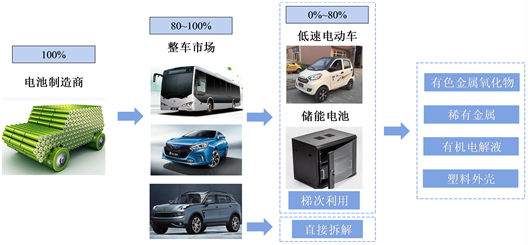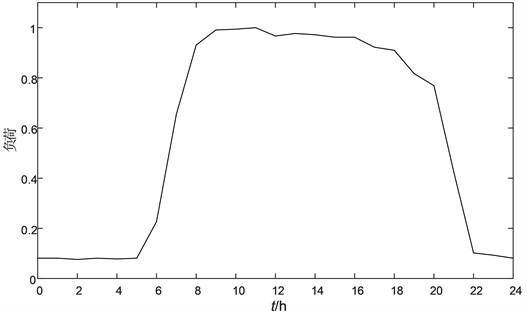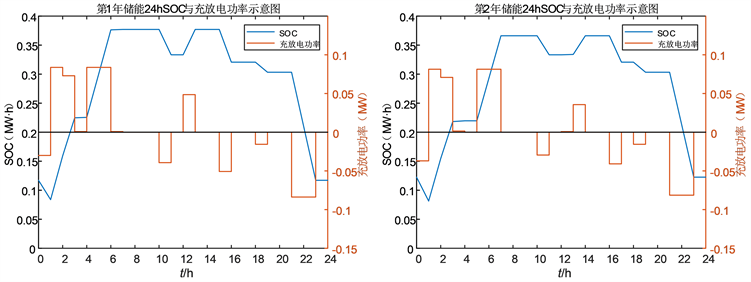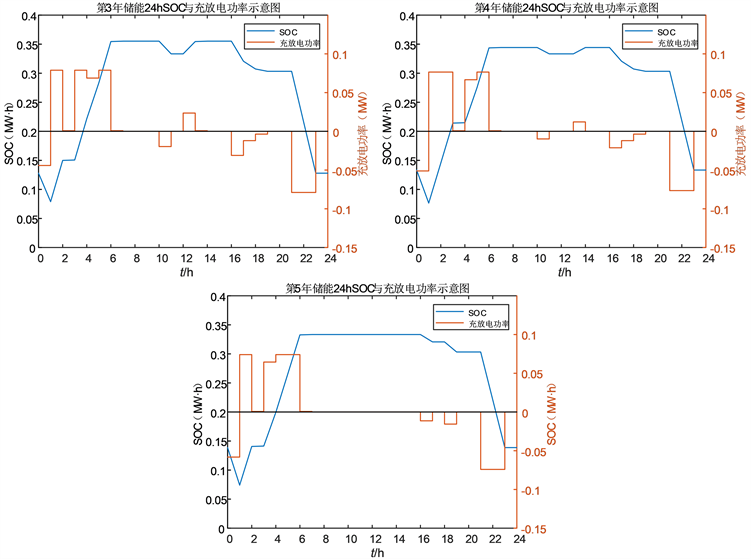﻿ 基于退役电池的用户侧储能备用电源配置方法

# 基于退役电池的用户侧储能备用电源配置方法Configuration Method of User-Side Energy Storage Backup Power Supply Based on Retired Batteries

Abstract: Retired power batteries still have a certain capacity and can still be used as energy storage on the user side. Retired batteries are used in the user-side energy storage system step by step, which can effectively improve the service life of power batteries, reduce the cost of energy storage system, improve resource utilization and maximize the value of power batteries. In the planning stage, the influence of battery capacity retention rate and cycle life is considered, the supporting role of battery energy storage system as backup power supply to load is considered and compared with the cost of diesel generator. The objective function is to maximize the economic benefit of the whole life cycle of energy storage device. A mixed integer linear programming model for the configuration of user-side energy storage backup power supply based on retired batteries was constructed. Taking a commercial user as an example, the user-side energy storage backup power configuration method based on retired batteries has significant economic benefits, which verifies the feasibility and effectiveness of the proposed method.

1. 引言

2. 退役电池梯次利用寿命分析Figure 1. Life cycle value diagram of power battery

$\beta =-2.6043\ast {10}^{-5}\ast N+0.8347$ (1)

${T}_{r}^{ESS}=\frac{{N}_{2}-{N}_{1}}{365\ast {N}_{peak}}$ (2)

${C}_{r}^{ESS}={C}^{ESS}\cdot \frac{{T}_{r}^{ESS}}{{T}^{ESS}}$ (3)

3. 基于退役电池的用户侧储能备用电源配置方法

3.1. 目标函数

$F=\underset{y=1}{\overset{Y}{\sum }}{\left(\frac{1+{i}_{r}}{1+{d}_{r}}\right)}^{y}\left({F}_{y,pl}^{ESS}+{F}_{loss}\right)-{F}_{inv}^{ESS}-\underset{y=1}{\overset{Y}{\sum }}{\left(\frac{1+{i}_{r}}{1+{d}_{r}}\right)}^{y}{F}_{ope}^{ESS}-{F}_{b}^{DG}-\underset{y=1}{\overset{Y}{\sum }}{\left(\frac{1+{i}_{r}}{1+{d}_{r}}\right)}^{y}{F}^{fuel}$ (4)

${F}_{y,pl}^{ESS}=365\underset{t=1}{\overset{24}{\sum }}{P}_{t}\cdot \left(-{p}_{y,t}^{ch}+{p}_{y,t}^{dis}\right)$ (5)

${F}_{loss}=\underset{t=1}{\overset{T}{\sum }}{P}_{pu,t}\cdot {P}_{loss,t}$ (6)

${L}_{olp}=\frac{\underset{t=1}{\overset{T}{\sum }}{P}_{loss,t}}{\underset{t=1}{\overset{T}{\sum }}{P}_{load,t}}$ (7)

${F}_{inv}^{ESS}=\left(1-\gamma \right)\cdot {C}_{r}^{ESS}\cdot {S}^{ESS}$ (8)

${F}_{ope}^{ESS}={c}^{ope}\cdot {C}_{r}^{ESS}\cdot {S}^{ESS}$ (9)

${f}_{\text{DE}}=\alpha {P}_{\text{DE}}+\beta {P}_{\text{DE},\text{rated}}$ (10)

${F}_{b}^{DG}={N}_{DG}\cdot {P}_{DG}$ (11)

${F}^{fuel}={C}_{fuel}\cdot {f}_{fuel}+{E}_{DG}\cdot \underset{w=1}{\overset{W}{\sum }}{P}_{w}\cdot {G}_{w}$ (12)

3.2. 约束条件

1) 充放电功率约束

$0\le {p}_{y,t}^{ch}\le {P}_{\mathrm{max}}$ (13)

$0\le {p}_{y,t}^{dis}\le {P}_{\mathrm{max}}$ (14)

2) 充放电守恒约束

$\underset{t=1}{\overset{24}{\sum }}{p}_{y,t}^{ch}=\underset{t=1}{\overset{24}{\sum }}{p}_{y,t}^{dis}$ (15)

3) 荷电状态约束

$SO{C}_{y,0}^{ESS}=SO{C}_{y,24}^{ESS}$ (16)

$0.1\cdot SO{C}_{\mathrm{max},y}\le SO{C}_{y,t}^{ESS}\le 0.9\cdot SO{C}_{\mathrm{max},y}$ (17)

${c}_{y,t}+{d}_{y,t}\le 1$ (18)

$SO{C}_{y,t}^{ESS}+\left({\eta }_{\text{ch}}\cdot {p}_{y,t}^{ch}-\frac{{p}_{y,t}^{dis}}{{\eta }_{\text{dis}}}\right)\Delta t=SO{C}_{y,t+1}^{ESS}$ (19)

$SO{C}_{y,t}^{ESS}\ge {T}_{s}\cdot {P}_{load,t}$ (20)

$SO{C}_{\mathrm{max},y}={S}^{ESS}\cdot \frac{{\beta }_{y}}{{\beta }_{st}}$ (21)

3.3. 规划模型及求解

max (4)

s.t. 式(13)-式(21)

4. 算例分析Figure 2. Typical commercial load curveTable 1. Time-of-use tariff for customersTable 2. Main parameters of cascade battery storage system and diesel generatorTable 3. Pollutant discharge or emission, pollution control standardsFigure 3. Diagram of SOC and charge-discharge power in retired battery life cycle

5. 总结

 王秉刚. 中国清洁汽车行动的成就与展望[J]. 汽车工程, 2005, 27(6): 643-647.

 Neubauer, J., Smith, K., Wood, E., et al. (2015) Identifyingand Overcoming Critical Barriers to Widespread Second Use of PEV Batteries. National Renewable Energy Lab (NREL), Golden.

 刘念, 唐霄, 段帅, 等. 考虑动力电池梯次利用的光伏换电站容量优化配置方法[J]. 中国电机工程学报, 2013, 33(4): 34-44+6.

 刘坚. 电动汽车退役电池储能应用潜力及成本分析[J]. 储能科学与技术, 2017, 6(2): 243-249.

 李建林, 修晓青, 刘道坦, 等. 计及政策激励的退役动力电池储能系统梯次应用研究[J]. 高电压技术, 2015, 41(8): 2562-2568.

 吴鸣, 孙丽敬, 寇凌峰, 等. 考虑需求侧响应的主动配电网电池梯次储能的容量配置方法[J]. 高电压技术, 2020, 46(1): 71-79.

 王帅, 尹忠东, 田硕文, 等. 基于退役动力电池的家庭储能容量优化配置[J]. 电测与仪表, 2020, 57(9): 58-64+88.

 韩晓娟, 张婳, 修晓青, 李建林. 配置梯次电池储能系统的快速充电站经济性评估[J]. 储能科学与技术, 2016, 5(4): 514-521.

 许欣慧, 舒征宇, 李世春. 基于退役电池在多储能场景下梯级利用的经济运行研究[J]. 智慧电力, 2020, 48(12): 58-64.

 李文超, 童亦斌, 张维戈. 计及电池使用寿命的电动汽车充电站储能容量配置方法[J]. 电工电能新技术, 2019, 39(4): 55-63.

 尚龙龙, 魏碧桧, 王伟, 等. 主动配电网储能动态配置规划方法[J]. 电力系统保护与控制, 2020, 48(17): 84-92.

 张婳. 计及梯次利用电池的储能优化规划[D]: [硕士学位论文]. 北京: 华北电力大学, 2017.

 邵志芳, 赵强, 张玉琼. 独立型微电网源荷协调配置优化[J/OL]. 电网技术, 1-12.
https://doi.org/10.13335/j.1000-3673.pst.2020.2028, 2021-09-24.

Top